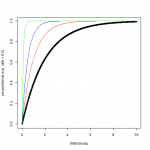Want to share your content on R-bloggers? click here if you have a blog, or here if you don't.In this set of exercises, we are going to explore some of the probability functions in R by using practical applications. Basic probability knowledge is required. In case you are not familiarized with the function `apply`, check the R documentation.

Note: We are going to use random numbers functions and random processes functions in R such as `runif`. A problem with these functions is that every time you run them, you will obtain a different value. To make your results reproducible you can specify the value of the seed using `set.seed(‘any number’)` before calling a random function. (If you are not familiar with seeds, think of them as the tracking number of your random number process.) For this set of exercises, we will use `set.seed(1).`Don’t forget to specify it before every exercise that includes random numbers.

Answers to the exercises are available here. If you obtained a different (correct) answer than those listed on the solutions page, please feel free to post your answer as a comment on that page.

Exercise 1

Generating dice rolls Set your seed to 1 and generate 30 random numbers using `runif`. Save it in an object called `random_numbers`. Then use the `ceiling` function to round the values. These values represent rolling dice values.

Exercise 2

Simulate one dice roll using the function `rmultinom`. Make sure `n = 1` is inside the function, and save it in an object called `die_result`. The matrix `die_result` is a collection of 1 one and 5 zeros, with the one indicating which value was obtained during the process. Use the function `which`to create an output that shows only the value obtained after the dice is rolled.

Exercise 3

Using `rmultinom`, simulate 30 dice rolls. Save it in a variable called `dice_result` and use `apply` to transform the matrix into a vector with the result of each dice.

Exercise 4

Some gambling games use 2 dice, and after being rolled they sum their value. Simulate throwing 2 dice 30 times and record the sum of the values of each pair. Use `rmultinom`to simulate throwing 2 dice 30 times. Use the function `apply` to record the sum of the values of each experiment.

Learn more about probability functions in the online course Statistics with R – Advanced Level. In this course you will learn how to

• work with different binomial and logistic regression techniques,
• know how to compare regression models and choose the right fit,
• and much more.

Exercise 5

Simulate normal distribution values. Imagine a population in which the average height is 1.70 m with a standard deviation of 0.1. Using `rnorm`, simulate the height of 100 people and save it in an object called `heights`.

To get an idea of the values of heights, use the function `summary`.

Exercise 6

90% of the population is smaller than ____________?

Exercise 7

Which percentage of the population is bigger than 1.60 m?

Exercise 8

Run the following line code before this exercise. This will load a library required for the exercise.

```if (!'MASS' %in% installed.packages()) install.packages('MASS') library(MASS)```

Simulate 1000 people with height and weight using the function `mvrnorm` with ` mu = c(1.70, 60) ` and
` Sigma = matrix(c(.1,3.1,3.1,100), nrow = 2) `

Exercise 9

How many people from the simulated population are taller than 1.70 m and heavier than 60 kg?

Exercise 10

How many people from the simulated population are taller than 1.75 m and lighter than 60 kg?